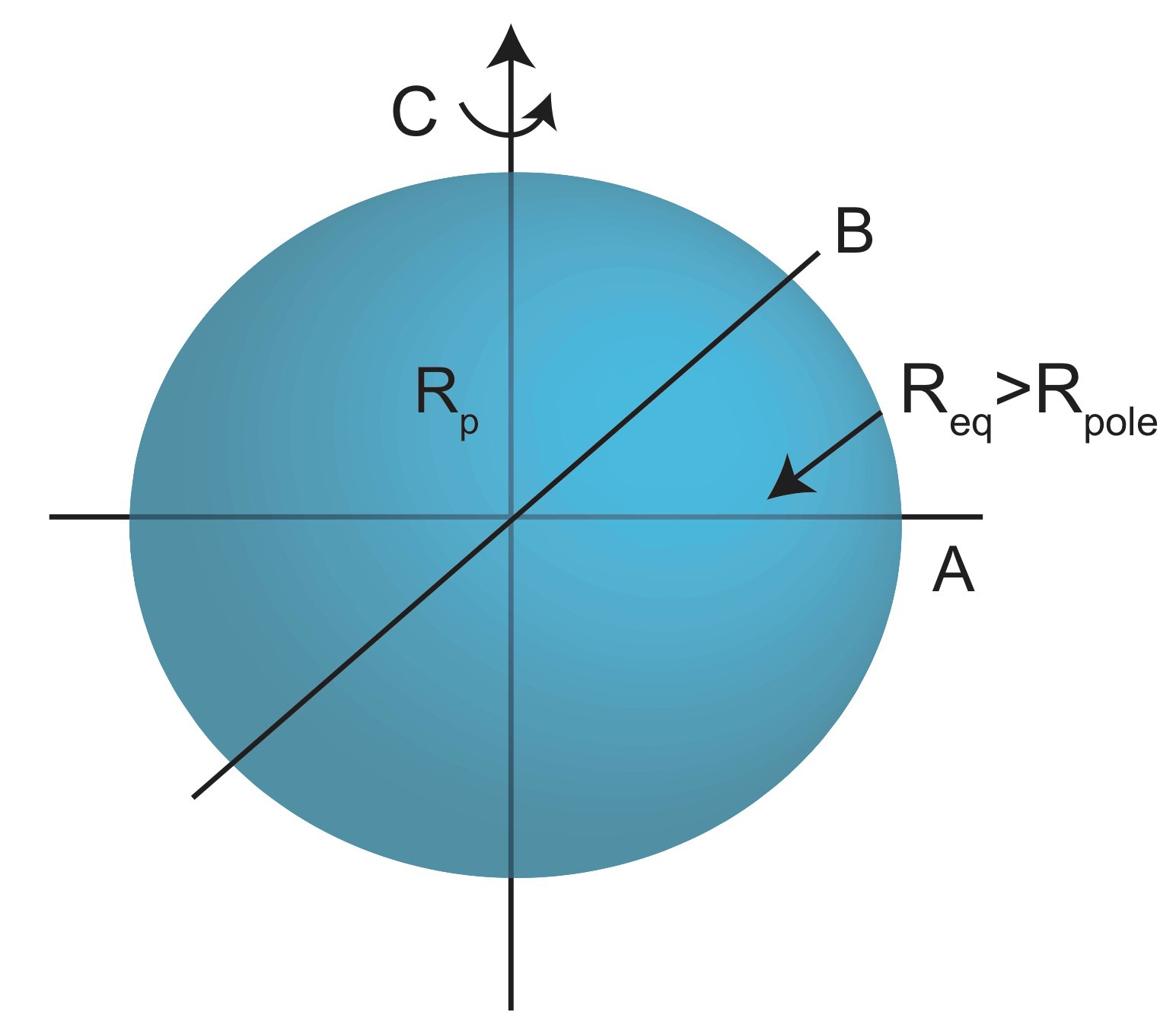# 3.2: Moments of Inertia

Now that we've covered some planetary motion and mass basics, let's move on to planetary structure. Knowing the mass of a planet is the first step to learning more about its internal structure. Information gathering about the particular body you want to study can be divided into three orders of knowledge:

• 1st Order: Mass→radius(and volume)→bulk/average density $$\rho=\frac{m}{v}$$
• 2nd Order: Layers (stratification)→Rm, Rc→Rplanet,$$\;\rho_m,\rho_c\rightarrow\rho_{bulk}$$→more layers
• 3rd Order: Internal (non-layered) density anomalies→+$$\Delta P$$(cold, and/or composition), -$$\Delta P$$(hot, and/or composition).Figure $$\PageIndex{1}$$: Planetary Structure

Layered structures have moments of Inertia.Figure $$\PageIndex{2}$$: Moments of Inertia

$I_P=\sum_{i=1}^{N} m_i{r_1}^2$

where mi is the point masses and r12 is the perpendicular distance from axis to the point mass.Figure $$\PageIndex{3}$$: Non Uniform Sphere

When $$\rho_c>\rho_m$$, more mass is closer to the axis compared to a uniform sphere.

⇒Ilayered<Iuniform

0.4MR2⇒less than 0.4⇒smaller layer⇒large pc and smaller Rc, but limits on pc (max pc)=pure iron

Example Earth

pbulk=5550$$\frac{kg}{m^3}$$

$$\frac{c}{ma^2}$$=0.33

Model 1 pm=4000$$\frac{kg}{m^3}$$, pc=10800$$\frac{kg}{m^3}$$, $$\frac{r_c}{r_m}$$=.06

Model 2 pm=2850$$\frac{kg}{m^3}$$, pc=8120$$\frac{kg}{m^3}$$, $$\frac{r_c}{r_m}$$=.08

## Ellipsoidal Bodies

It is important to remember that Earth, and planets in general, are not spheres, they are ellipsoids.Figure $$\PageIndex{4}$$: Ellipsoidal Body

C=axial moment of inertia

A&B=equatorial moment of inertia

Req>Rpole

C>B, A

A$$\approx$$B

If we knew C (and A, B), we could use m, R, and pbulk to estimate the internal layered structure (p and Rs trade off). Add psurface, composition to improve estimate (lockdown pm).

## Determining Moment of Inertia for Planets

If we know the moment of inertia of a planet and its mass, it is possible to determine simple models of the layered density structure of the planet. The layered density structure is defined by the thickness of the layers and the density of each layer. Usually, it is possible to determine reasonable 2-layered structures with additional information about the composition of density at the surface.

Procedure for Determining Moment of Inertia

The main assumption in the following procedure is that the planet behaves like a fluid over long periods of time. This is called the hydrostatic assumption. This is a reasonable assumption for rocky planets, which have mantle compositions similar to the earth.

• The flattening of a rotating, fluid planet, with a polar radius, c, and equatorial radius, a, is given by:

$f=\frac{a-c}{a}$

The equatorial radius is larger because the spinning motion of the planet (centripetal force) causes mass to move to the equator and away from the poles. The flattening can be determined by measuring the gravitational potential field, through the orbit of satellites. The orbit will be perpetuated by the non-spherical potential field, and these perturbations can be related to the actual shape of the gravitational potential.

• The flattening of a hydrostatic planet can be written in different ways. We will use two different ways to allow us to combine them in order to determine the moment of inertia. First,

$f_{hyd}\approx\frac{3}{2}J_2+\frac{1}{2}\frac{a^3\omega^2}{2GM}$

where J2=$$\frac{C-A}{Ma^2}$$ is the ellipticity coefficient (one of the terms in the spherical harmonic representation of the gravitational potential field). All of the information in this equation can be observed/measured.

• The flattening can also be determined by an approximate theory of the response of the planet to its own rotation, called the Darwin-Radau Approximation:

$f_{hyd}\approx\frac{\frac{5}{2}\frac{a^3\omega^2}{GM}}{1+\frac{25}{4}(1-\frac{3}{2}\frac{C}{Ma^2})^2}$

In this equation, everything can be measured/observed except for the moment of inertia $$\frac{C}{Ma^2}$$

• The moment of inertia can be determined by solving the Darwin Radau equation for the moment of inertia, and using equation 3.2.3 to calculate fhyd:

$\frac{C}{Ma^2}\approx\frac{2}{3}(1-\sqrt{\frac{2}{5}\frac{\frac{a^3\omega^2}{GM}}{f_{hyd}}-\frac{4}{25}})$